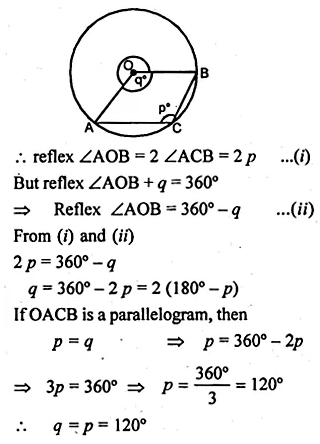Guru

# Question 9 (b) In the figure (ii) given below, C is a point on the minor arc AB of the circle with centre O. Given ∠ACB = p°, ∠AOB = q°, express q in terms of p. Calculate p if OACB is a parallelogram.

• 1

This is the basic and conceptual question from Chapter name- circles
Topic – Angle properties of circles
Chapter number- 15

We have been given the figure of circle with certain information and we have to find <ACB, <AOB in terms of p and q and also we have to prove that OACB is parallelogram.

ICSE Avichal publication
Understanding ICSE Mathematics
Question 9(b)

Share

1.